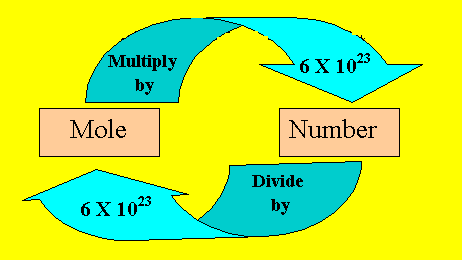The mole

just like a DOZEN, only bigger

The mole is the number 6.02 X 1023. It is also the mass of substance that contains this number of units of the substance. A big number by any standards, but one that is necessary when we consider the size of the molecules and atoms we are trying to deal with. This number is called Avogadro's number. Atoms and molecules are so small, that it is impossible to measure the mass of one million or even one billion of these tiny particles. So we measure them in lots of 6.02 X 1023. We can effectively compare the dozen to the mole. Instead of using the number 12, as we do in dozens, we use the number 6.02 X 1023.

Complete the table below.